# Early View of the Universe

27 de Feb de 2012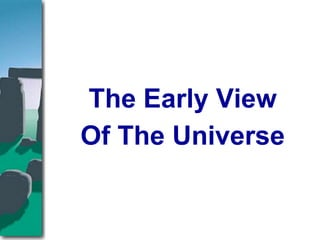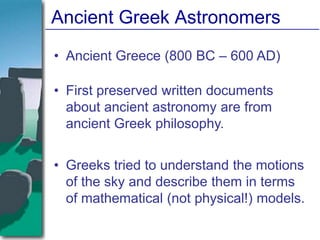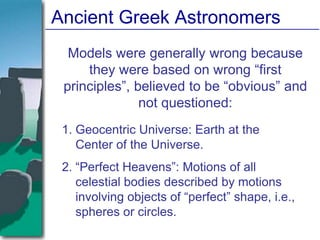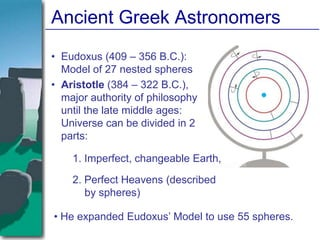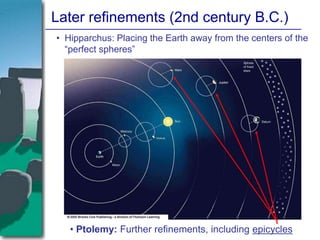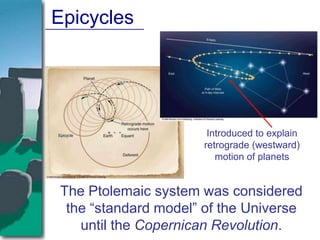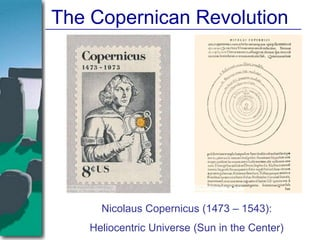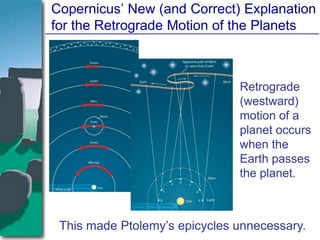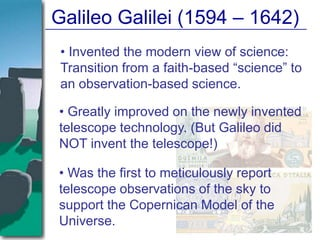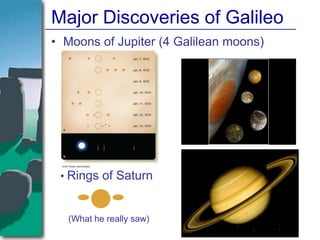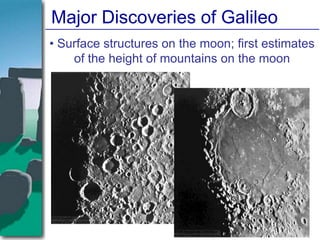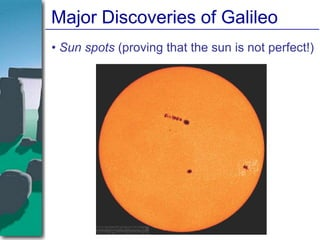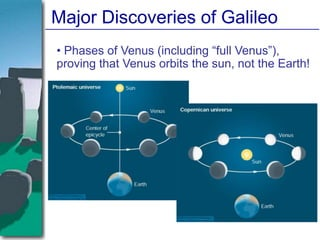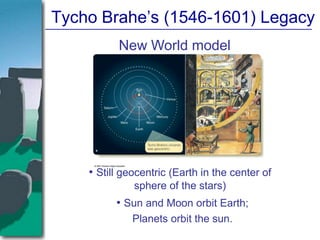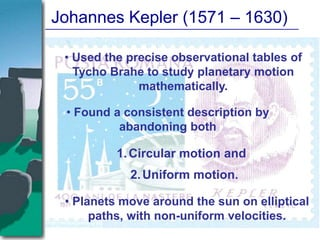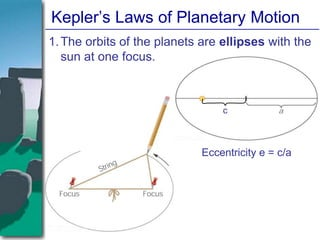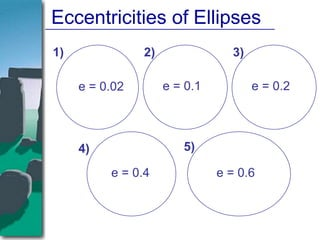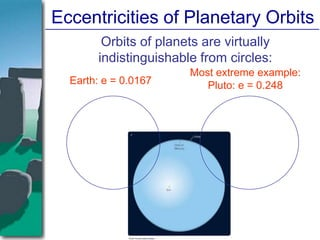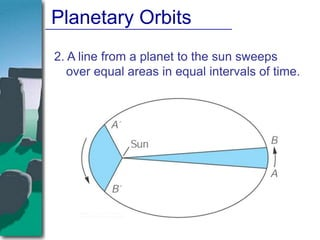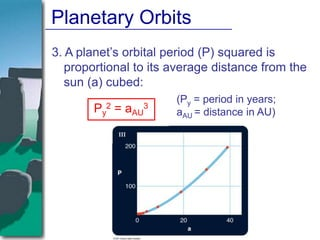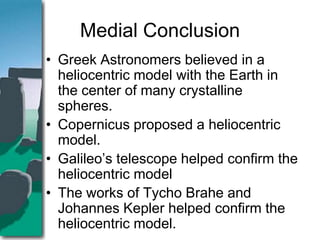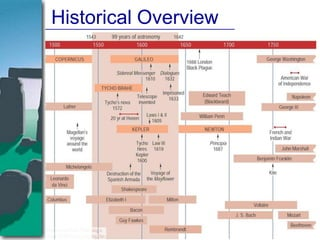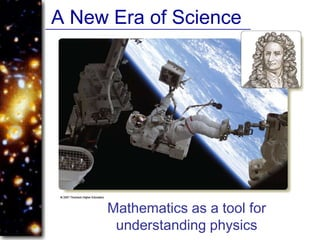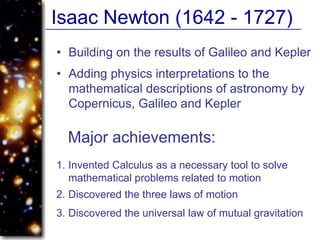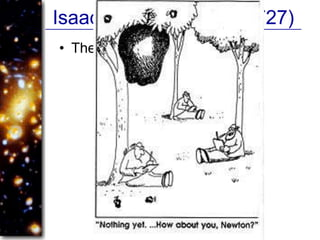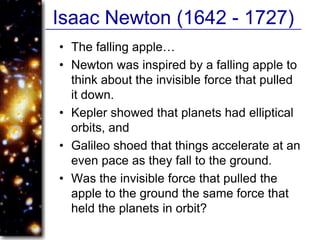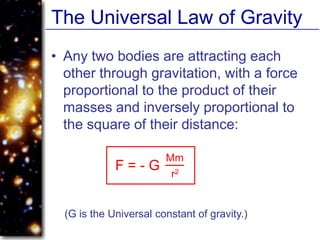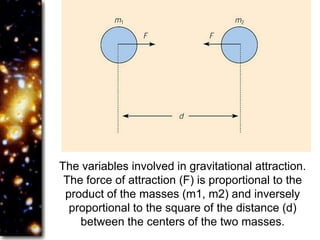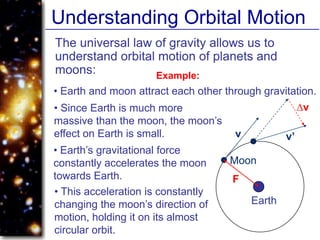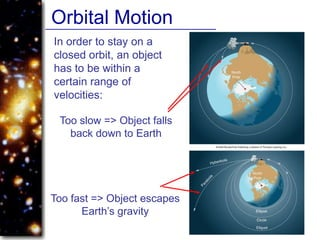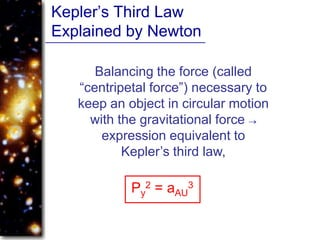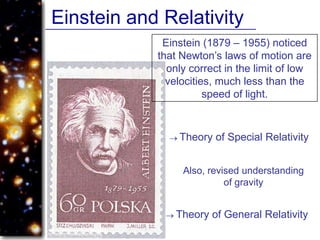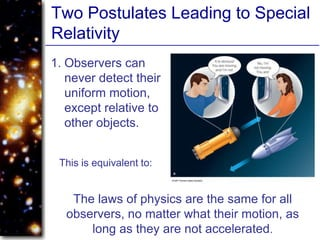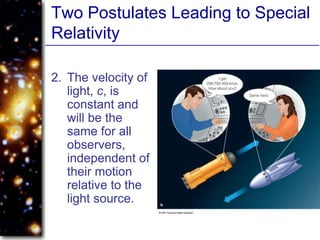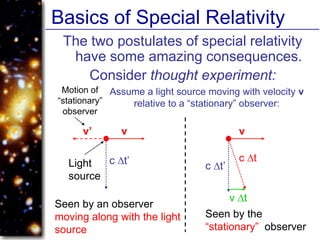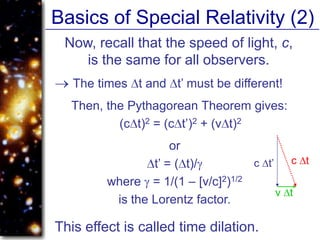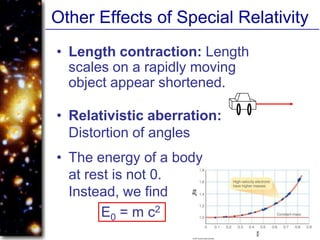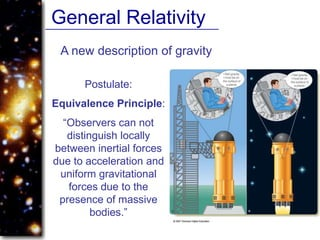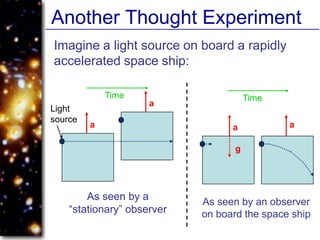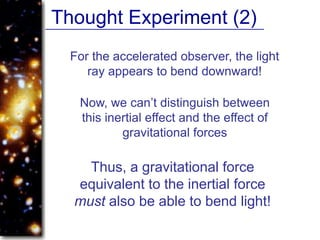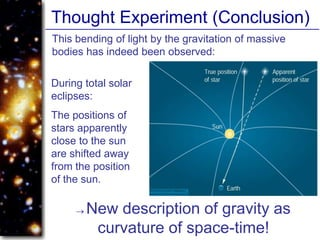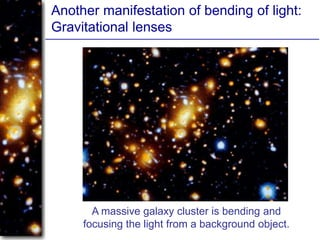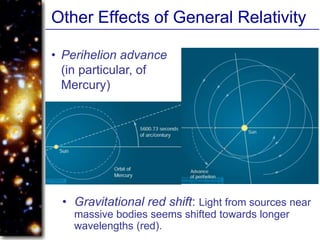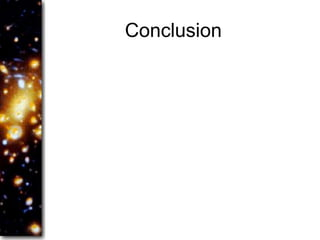1 de 44

### Early View of the Universe

• 1. The Early View Of The Universe
• 2. Ancient Greek Astronomers • Ancient Greece (800 BC – 600 AD) • First preserved written documents about ancient astronomy are from ancient Greek philosophy. • Greeks tried to understand the motions of the sky and describe them in terms of mathematical (not physical!) models.
• 3. Ancient Greek Astronomers Models were generally wrong because they were based on wrong “first principles”, believed to be “obvious” and not questioned: 1. Geocentric Universe: Earth at the Center of the Universe. 2. “Perfect Heavens”: Motions of all celestial bodies described by motions involving objects of “perfect” shape, i.e., spheres or circles.
• 4. Ancient Greek Astronomers • Eudoxus (409 – 356 B.C.): Model of 27 nested spheres • Aristotle (384 – 322 B.C.), major authority of philosophy until the late middle ages: Universe can be divided in 2 parts: 1. Imperfect, changeable Earth, 2. Perfect Heavens (described by spheres) • He expanded Eudoxus’ Model to use 55 spheres.
• 5. Later refinements (2nd century B.C.) • Hipparchus: Placing the Earth away from the centers of the “perfect spheres” • Ptolemy: Further refinements, including epicycles
• 6. Epicycles Introduced to explain retrograde (westward) motion of planets The Ptolemaic system was considered the “standard model” of the Universe until the Copernican Revolution.
• 7. The Copernican Revolution Nicolaus Copernicus (1473 – 1543): Heliocentric Universe (Sun in the Center)
• 8. Copernicus’ New (and Correct) Explanation for the Retrograde Motion of the Planets Retrograde (westward) motion of a planet occurs when the Earth passes the planet. This made Ptolemy’s epicycles unnecessary.
• 9. Galileo Galilei (1594 – 1642) • Invented the modern view of science: Transition from a faith-based “science” to an observation-based science. • Greatly improved on the newly invented telescope technology. (But Galileo did NOT invent the telescope!) • Was the first to meticulously report telescope observations of the sky to support the Copernican Model of the Universe.
• 10. Major Discoveries of Galileo • Moons of Jupiter (4 Galilean moons) • Rings of Saturn (What he really saw)
• 11. Major Discoveries of Galileo • Surface structures on the moon; first estimates of the height of mountains on the moon
• 12. Major Discoveries of Galileo • Sun spots (proving that the sun is not perfect!)
• 13. Major Discoveries of Galileo • Phases of Venus (including “full Venus”), proving that Venus orbits the sun, not the Earth!
• 14. Tycho Brahe’s (1546-1601) Legacy New World model • Still geocentric (Earth in the center of sphere of the stars) • Sun and Moon orbit Earth; Planets orbit the sun.
• 15. Johannes Kepler (1571 – 1630) • Used the precise observational tables of Tycho Brahe to study planetary motion mathematically. • Found a consistent description by abandoning both 1. Circular motion and 2. Uniform motion. • Planets move around the sun on elliptical paths, with non-uniform velocities.
• 16. Kepler’s Laws of Planetary Motion 1. The orbits of the planets are ellipses with the sun at one focus. c Eccentricity e = c/a
• 17. Eccentricities of Ellipses 1) 2) 3) e = 0.02 e = 0.1 e = 0.2 4) 5) e = 0.4 e = 0.6
• 18. Eccentricities of Planetary Orbits Orbits of planets are virtually indistinguishable from circles: Most extreme example: Earth: e = 0.0167 Pluto: e = 0.248
• 19. Planetary Orbits 2. A line from a planet to the sun sweeps over equal areas in equal intervals of time.
• 20. Planetary Orbits 3. A planet’s orbital period (P) squared is proportional to its average distance from the sun (a) cubed: (Py = period in years; Py2 = aAU3 aAU = distance in AU)
• 21. Medial Conclusion • Greek Astronomers believed in a heliocentric model with the Earth in the center of many crystalline spheres. • Copernicus proposed a heliocentric model. • Galileo’s telescope helped confirm the heliocentric model • The works of Tycho Brahe and Johannes Kepler helped confirm the heliocentric model.
• 23. A New Era of Science Mathematics as a tool for understanding physics
• 24. Isaac Newton (1642 - 1727) • Building on the results of Galileo and Kepler • Adding physics interpretations to the mathematical descriptions of astronomy by Copernicus, Galileo and Kepler Major achievements: 1. Invented Calculus as a necessary tool to solve mathematical problems related to motion 2. Discovered the three laws of motion 3. Discovered the universal law of mutual gravitation
• 25. Isaac Newton (1642 - 1727) • The falling apple…
• 26. Isaac Newton (1642 - 1727) • The falling apple… • Newton was inspired by a falling apple to think about the invisible force that pulled it down. • Kepler showed that planets had elliptical orbits, and • Galileo shoed that things accelerate at an even pace as they fall to the ground. • Was the invisible force that pulled the apple to the ground the same force that held the planets in orbit?
• 27. The Universal Law of Gravity • Any two bodies are attracting each other through gravitation, with a force proportional to the product of their masses and inversely proportional to the square of their distance: Mm F=-G r2 (G is the Universal constant of gravity.)
• 28. The variables involved in gravitational attraction. The force of attraction (F) is proportional to the product of the masses (m1, m2) and inversely proportional to the square of the distance (d) between the centers of the two masses.
• 29. Understanding Orbital Motion The universal law of gravity allows us to understand orbital motion of planets and moons: Example: • Earth and moon attract each other through gravitation. • Since Earth is much more v massive than the moon, the moon’s effect on Earth is small. v v’ • Earth’s gravitational force constantly accelerates the moon Moon towards Earth. F • This acceleration is constantly changing the moon’s direction of Earth motion, holding it on its almost circular orbit.
• 30. Orbital Motion In order to stay on a closed orbit, an object has to be within a certain range of velocities: Too slow => Object falls back down to Earth Too fast => Object escapes Earth’s gravity
• 31. Kepler’s Third Law Explained by Newton Balancing the force (called “centripetal force”) necessary to keep an object in circular motion with the gravitational force expression equivalent to Kepler’s third law, Py2 = aAU3
• 32. Einstein and Relativity Einstein (1879 – 1955) noticed that Newton’s laws of motion are only correct in the limit of low velocities, much less than the speed of light. Theory of Special Relativity Also, revised understanding of gravity Theory of General Relativity
• 33. Two Postulates Leading to Special Relativity 1. Observers can never detect their uniform motion, except relative to other objects. This is equivalent to: The laws of physics are the same for all observers, no matter what their motion, as long as they are not accelerated.
• 34. Two Postulates Leading to Special Relativity 2. The velocity of light, c, is constant and will be the same for all observers, independent of their motion relative to the light source.
• 35. Basics of Special Relativity The two postulates of special relativity have some amazing consequences. Consider thought experiment: Motion of Assume a light source moving with velocity v “stationary” relative to a “stationary” observer: observer v’ v v c t’ c t Light c t’ source v t Seen by an observer moving along with the light Seen by the source “stationary” observer
• 36. Basics of Special Relativity (2) Now, recall that the speed of light, c, is the same for all observers. The times t and t’ must be different! Then, the Pythagorean Theorem gives: (c t)2 = (c t’)2 + (v t)2 or t’ = ( t)/ c t’ c t where = 1/(1 – [v/c]2)1/2 v t is the Lorentz factor. This effect is called time dilation.
• 37. Other Effects of Special Relativity • Length contraction: Length scales on a rapidly moving object appear shortened. • Relativistic aberration: Distortion of angles • The energy of a body at rest is not 0. Instead, we find E0 = m c2
• 38. General Relativity A new description of gravity Postulate: Equivalence Principle: “Observers can not distinguish locally between inertial forces due to acceleration and uniform gravitational forces due to the presence of massive bodies.”
• 39. Another Thought Experiment Imagine a light source on board a rapidly accelerated space ship: Time Time a Light source a a a g As seen by a As seen by an observer “stationary” observer on board the space ship
• 40. Thought Experiment (2) For the accelerated observer, the light ray appears to bend downward! Now, we can’t distinguish between this inertial effect and the effect of gravitational forces Thus, a gravitational force equivalent to the inertial force must also be able to bend light!
• 41. Thought Experiment (Conclusion) This bending of light by the gravitation of massive bodies has indeed been observed: During total solar eclipses: The positions of stars apparently close to the sun are shifted away from the position of the sun. New description of gravity as curvature of space-time!
• 42. Another manifestation of bending of light: Gravitational lenses A massive galaxy cluster is bending and focusing the light from a background object.
• 43. Other Effects of General Relativity • Perihelion advance (in particular, of Mercury) • Gravitational red shift: Light from sources near massive bodies seems shifted towards longer wavelengths (red).